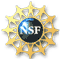# Randomizing parameters in a WeBWorK Problem

Part of the essence of a WeBWorK problem problem is that it normally contains random parameters. This is accomplished through use of the language perl with extra pieces provided by WeBWorK.

In perl, variables start with "\$". So, you can have variables such as "\$a", "\$hello5", "\$scooby_doo".

You may want to randomize one thing, and then compute others (e.g., compute initial parameters, and then compute something which goes into the answer). Sometimes, you might randomly pick the answer and then compute the other way to find out what goes into the question.

## The basic way to pick random numbers

Pick a random integer between 2 and 5 (inclusive), and put it in the variable `a1`:

```\$a1 = random(2, 5);
```

Pick a random number between 2 and 5 using steps of 0.1, and put it in the variable `a2`:

```\$a2 = random(2, 5, 0.1);
```

Pick a random integer between -2 and 5, but not zero (which might cause problems in the problem), and put it in the variable `leadingcoef`:

```\$leadingcoef = non_zero_random(-2,5);
```

As mentioned above, you might want to compute the answer at this point. If `\$a` and `\$b` contain the lengths of sides of a right triangle, we can put the length of the hypoteneuse in `\$c` with:

```\$c = sqrt(\$a**2 + \$b**2);
```

## Fancier Randomization

Suppose we have already picked one number \$a, and now we want to pick \$b from 1 to 10 so that it is not equal to \$a. The basic form is

```do { \$b = random(1, 10); } until (\$b != \$a);
```
This will keep picking random numbers until we have one we like. The condition for `until` can contain lots of conditions. For example, if we now want to pick \$c which is different from both \$a and \$b, we can use
```do { \$c = random(1, 10); } until ((\$c != \$a) and (\$c != \$b));
```

Sometimes, you might just want to pick from a list of numbers. For example, in a compound interest problem, you might want to stick to certain "realistic" numbers of months. Then we can use,

```\$months = list_random(3, 4, 6, 9, 15, 18);
```

It is also possible to pick fractions in lowest terms or make a list of things and shuffle their order, or pick some subset out of a list. These are not needed very often, so contact John for details if this sounds useful for a problem you are working on.

John JonesThe work represented here was produced with partial support from a grant by the National Science Foundation (DUE-0125369).